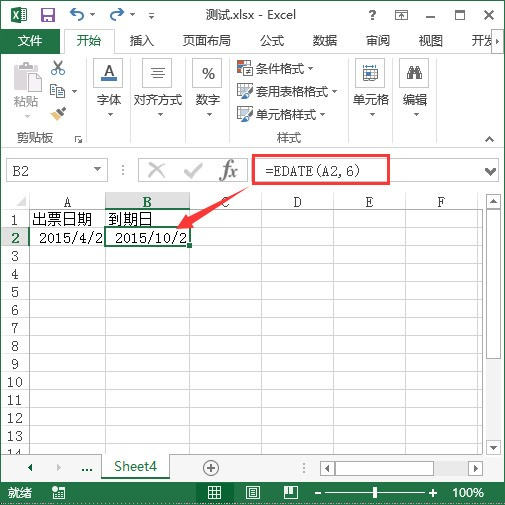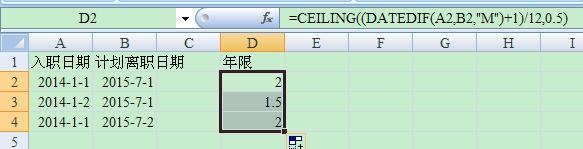• ZOL首页
• 产品报价
• 论坛
• ZOL问答
• 更多
• 手机客户端

Excel肿么计算两个日期间隔有多少个月

Excel肿么计算两个日期间隔有多少个月

• 回答数

7

• 浏览数

8,303

### 7个回答 默认排序 默认排序 按时间排序

=ROUNDDOWN(YEARFRAC(A2,B2)*12,0) 向下取整，不足一个月不计数。
=ROUNDUP(YEARFRAC(A1,B1)*12,0) 向上取整，不足一个月算一个月。
=ROUND(YEARFRAC(A1,B1)*12,0) 四舍五入，不足一个月的15天以下不计数，超过15天算一个月。

=DATE(YEAR(A2),MONTH(A2)+N,DAY(A2)) 公式中的N为你要增加的月值Excel版本参考：2013

1、B2单元格输入：=EDATE(A2,6)
2、回车

EDATE函数语法：EDATE(开始日期，月份）=DATE(YEAR(A1),MONTH(A1)+6,DAY(A1))

=CEILING((DATEDIF(A2,B2,"M")+1)/12,0.5)### 最新回答 更多>

ZOL问答 > Excel肿么计算两个日期间隔有多少个月

### 举报成功